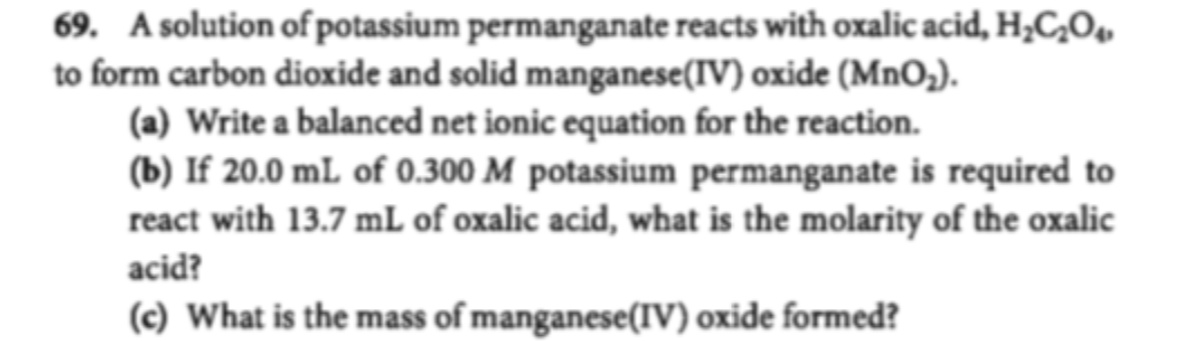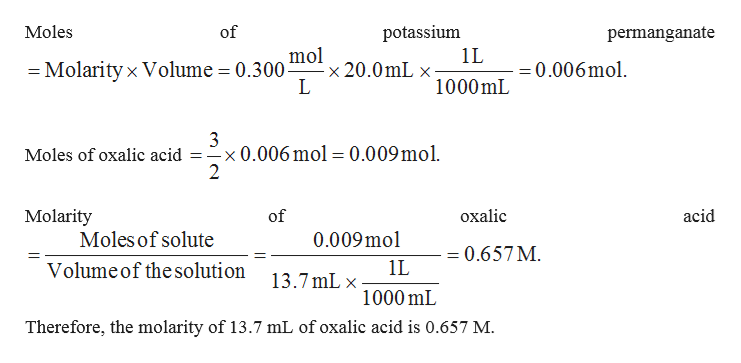# 69. A solution of potassium permanganate reacts with oxalic acid, H2C20to form carbon dioxide and solid manganese (IV) oxide (MnO2).(a) Write a balanced net ionic equation for the reaction(b) If 20.0 mL of 0.300 M potassium permanganate is required toreact with 13.7 mL of oxalic acid, what is the molarity of the oxalicacid?(c) What is the mass of manganese(IV) oxide formed?

Question
648 views

How to solve number 69? Please explain from the basic :)help_outlineImage Transcriptionclose69. A solution of potassium permanganate reacts with oxalic acid, H2C20 to form carbon dioxide and solid manganese (IV) oxide (MnO2). (a) Write a balanced net ionic equation for the reaction (b) If 20.0 mL of 0.300 M potassium permanganate is required to react with 13.7 mL of oxalic acid, what is the molarity of the oxalic acid? (c) What is the mass of manganese(IV) oxide formed? fullscreen
check_circle

Step 1

(a) A balanced net ionic equation for the reaction between potassium permanganate and oxalic acid to give carbon dioxide and solid manganese oxide is given below.

Step 2

(b) From the above balanced chemical equation, it can be stated that 2 moles of KMnO4 is reacting with 3 moles of oxalic acid to pro...help_outlineImage TranscriptioncloseMoles of potassium permanganate mol x 20.0mL x L 1L =0.006mol -Molarity x Volume = 0.300- 1000mL 3 Moles of oxalic acid = -x0.006 mol = 0.009mol 2 Molarity of охаlic acid Moles of solute 0.009mol =0.657M 1 1L Volumeof the solution 13.7mL x 1000 mL Therefore, the molarity of 13.7 mL of oxalic acid is 0.657 M. fullscreen

### Want to see the full answer?

See Solution

#### Want to see this answer and more?

Solutions are written by subject experts who are available 24/7. Questions are typically answered within 1 hour.*

See Solution
*Response times may vary by subject and question.
Tagged in

### Quantitative analysis